### Home > CALC > Chapter Ch10 > Lesson 10.3.1 > Problem10-114

10-114.
1. Evaluate the following limits. Homework Help ✎

1.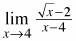2.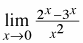3.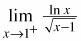4.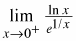5.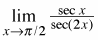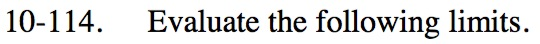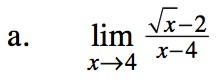The denominator can be factored as:

$(\sqrt{x}+2)(\sqrt{x}-2)$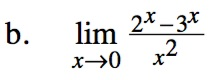Use l'Hôpital's Rule, but only once.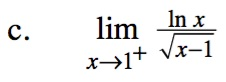Use l'Hôpital's Rule twice.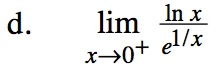$=\lim_{x\to 0^+}\frac{1/x}{e^{1/x}(-x^{-2})}$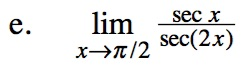Rewrite the argument of the limit as: